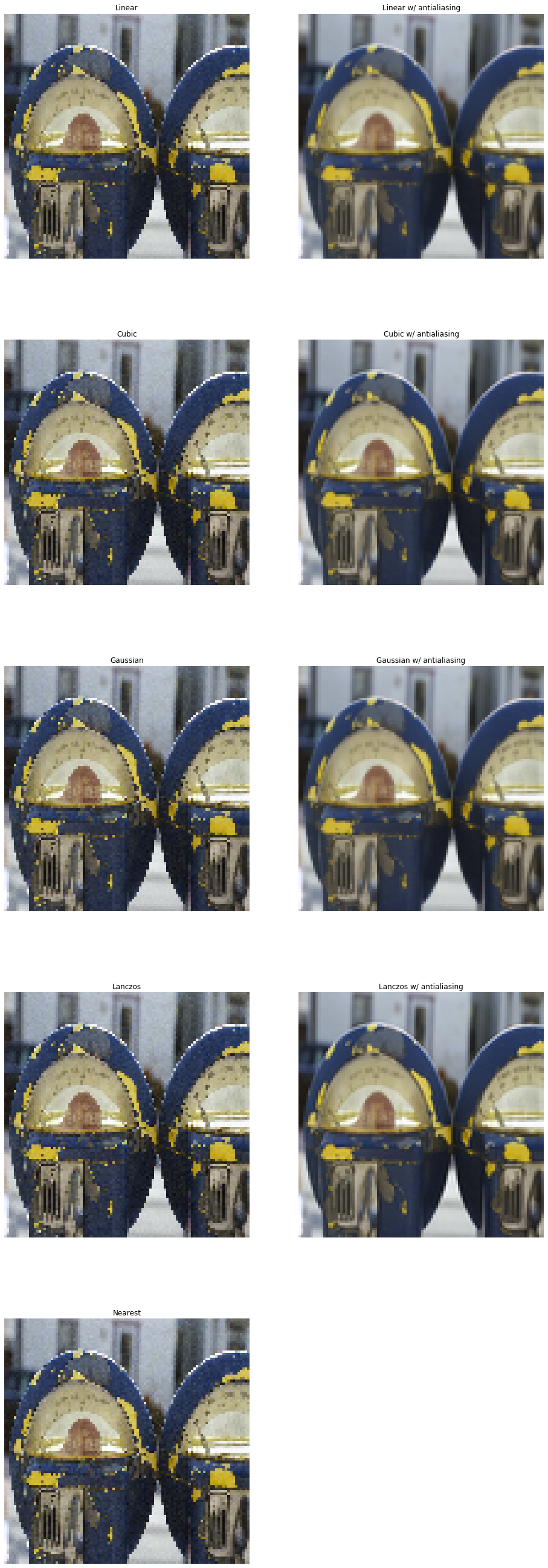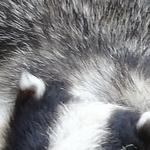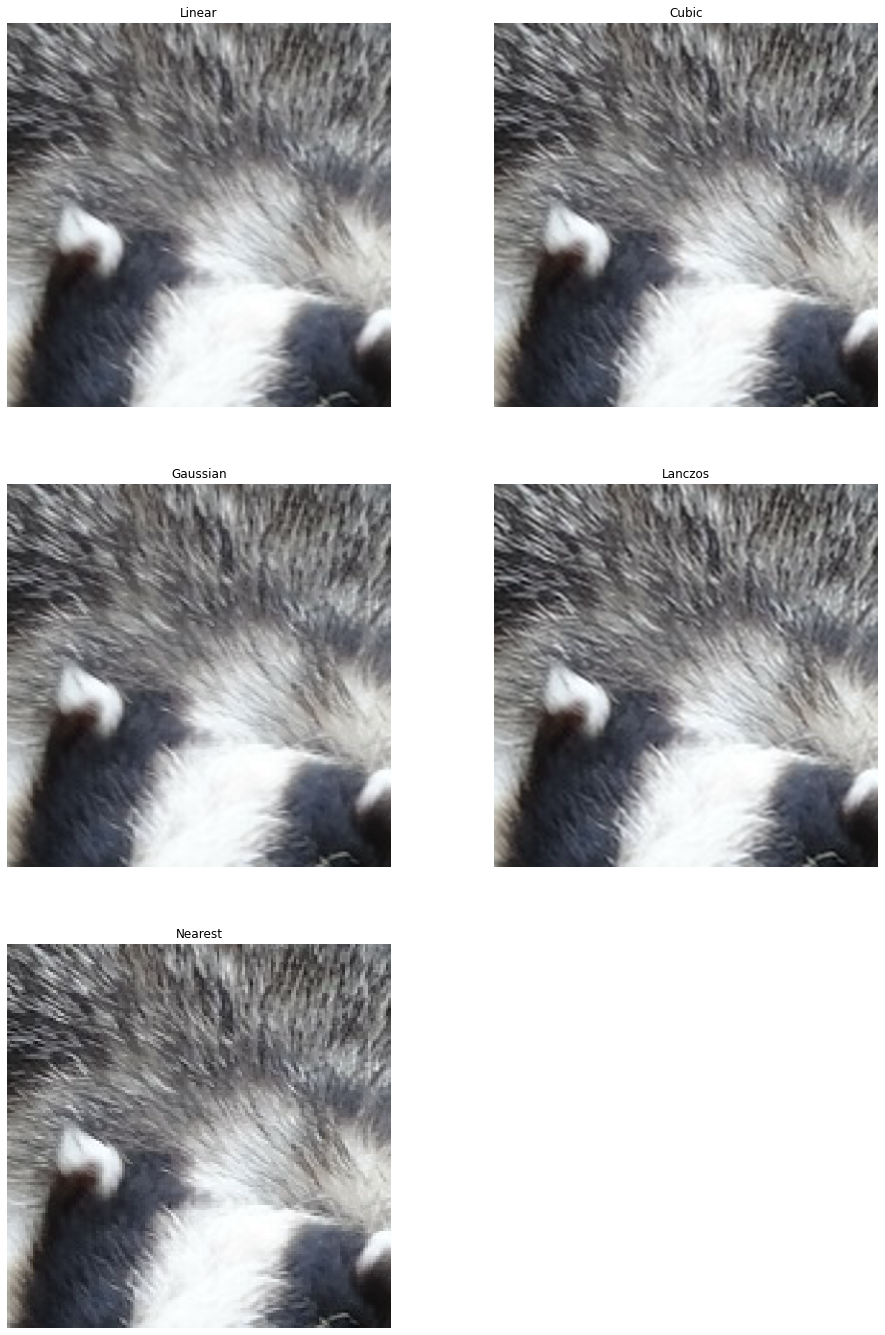# Interpolation methods¶

This example compares various interpolation methods available when resizing.

DALI_EXTRA_PATH environment variable should point to the place where data from DALI extra repository is downloaded. Please make sure that the proper release tag is checked out.

:

from nvidia.dali.pipeline import Pipeline
import nvidia.dali as dali
import numpy as np
import matplotlib.pyplot as plt
import math
import os.path
import PIL

test_data_root = os.environ['DALI_EXTRA_PATH']
db_folder = os.path.join(test_data_root, 'db', 'lmdb')


## Downscaling¶

First, let’s define a pipeline that dowscales images aggressively to a fixed size using different interpolation methods:

:

batch_size = 32

pipe = dali.pipeline.Pipeline(batch_size, 3, 0)
with pipe:
files, labels = dali.fn.readers.caffe(path = db_folder, random_shuffle = True, seed = 1234)
images = dali.fn.decoders.image(files, device = "mixed")

width = 100
height = 100

resize_outs =  (
dali.fn.resize(images, resize_x=width, resize_y=height, interp_type=interp, antialias=antialias)
for interp, antialias in [
(dali.types.INTERP_LINEAR, False),
(dali.types.INTERP_LINEAR, True),
(dali.types.INTERP_CUBIC, False),
(dali.types.INTERP_CUBIC, True),
(dali.types.INTERP_GAUSSIAN, False),
(dali.types.INTERP_GAUSSIAN, True),
(dali.types.INTERP_LANCZOS3, False),
(dali.types.INTERP_LANCZOS3, True),
(dali.types.INTERP_NN, False),
]
)

pipe.set_outputs(images, *resize_outs)

pipe.build()
pipe_out = pipe.run()


Let’s define a utility for diplaying the output images

:

def show(pipe_out, idx, methods, figsize=(16, 32)):
from synsets import imagenet_synsets
import matplotlib.gridspec as gridspec
len_outputs = len(pipe_out)
fig = plt.figure(figsize=figsize)
plt.suptitle(None)
columns = 2
rows = int(math.ceil(len_outputs / columns))
gs = gridspec.GridSpec(rows, columns)
for i in range(len_outputs):
plt.subplot(gs[i])
plt.axis("off")
plt.title(methods[i])
pipe_out_cpu = pipe_out[i].as_cpu()
img_chw = pipe_out_cpu.at(idx)
plt.imshow((img_chw)/255.0)

:

print("Original image")
PIL.Image.fromarray(pipe_out.as_cpu().at(5))

Original image

::

methods = ["Linear", "Linear w/ antialiasing",
"Cubic", "Cubic w/ antialiasing",
"Gaussian", "Gaussian w/ antialiasing",
"Lanczos", "Lanczos w/ antialiasing",
"Nearest"]
show(pipe_out[1:], 5, methods, figsize=(16,48))As we can clearly see, interpolations without anti-aliasing are not suitable for aggreesive downscaling.

## Upscaling¶

Now, let’s compare how the interpolation methods fare at upscaling. For good visualization, we’ll take a small crop of the input.

:

batch_size = 32

pipe = dali.pipeline.Pipeline(batch_size, 3, 0)
with pipe:
files, labels = dali.fn.readers.caffe(path = db_folder, random_shuffle = True, seed = 1234)
images = dali.fn.decoders.image(files, device = "mixed")

width = 400
height = 400

cropped = dali.fn.crop(images, crop=(150,150))

nearest, linear, cubic, gaussian, lanczos =  (
dali.fn.resize(cropped, resize_x=width, resize_y=height, interp_type=interp)
for interp in [
dali.types.INTERP_LINEAR,
dali.types.INTERP_CUBIC,
dali.types.INTERP_GAUSSIAN,
dali.types.INTERP_LANCZOS3,
dali.types.INTERP_NN,
]
)

pipe.set_outputs(cropped, nearest, linear, cubic, gaussian, lanczos)

pipe.build()
pipe_out = pipe.run()

[/home/janton/git/dali/dali/operators/image/resize/resampling_attr.cc:97] The default behavior for LINEAR interpolation type has been changed to apply an antialiasing filter. If you didn't mean to apply an antialiasing filter, please use antialias=False

:

print("Original image")
PIL.Image.fromarray(pipe_out.as_cpu().at(1))

Original image

::

methods = ["Linear", "Cubic", "Gaussian", "Lanczos", "Nearest"]
show(pipe_out[1:], 1, methods, figsize=(16, 24))As expected, nearest neighbor interpolation produces severe block artifacs. More sophisticated filters produce far better results, but require more computational power.